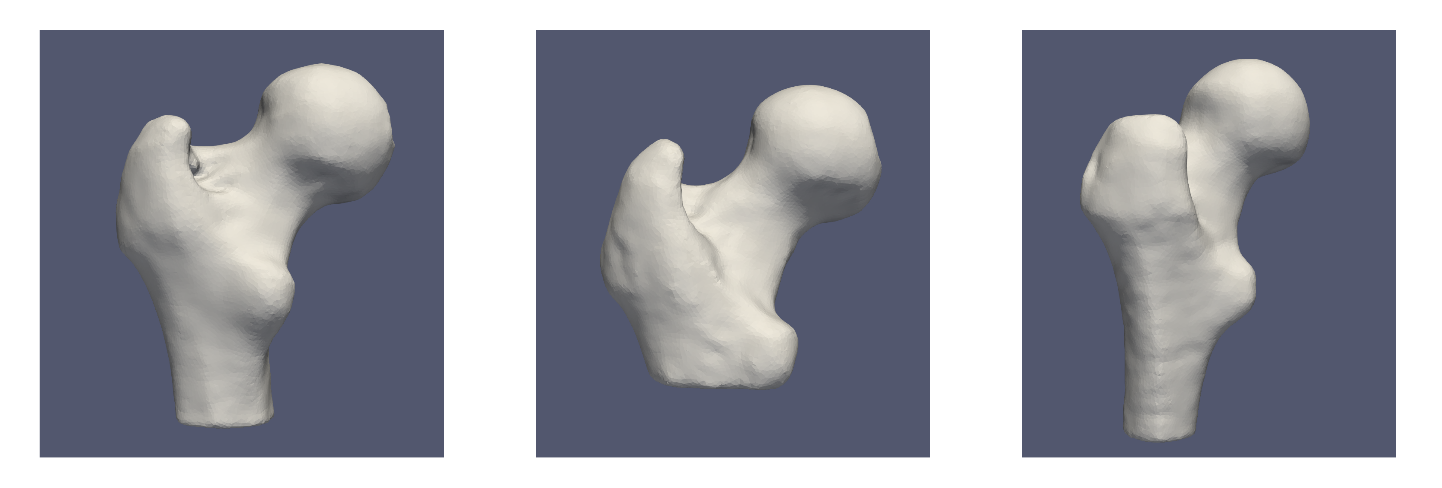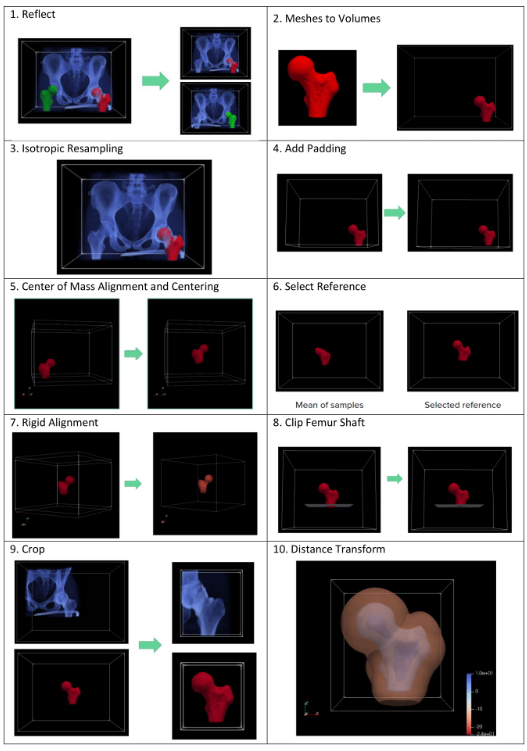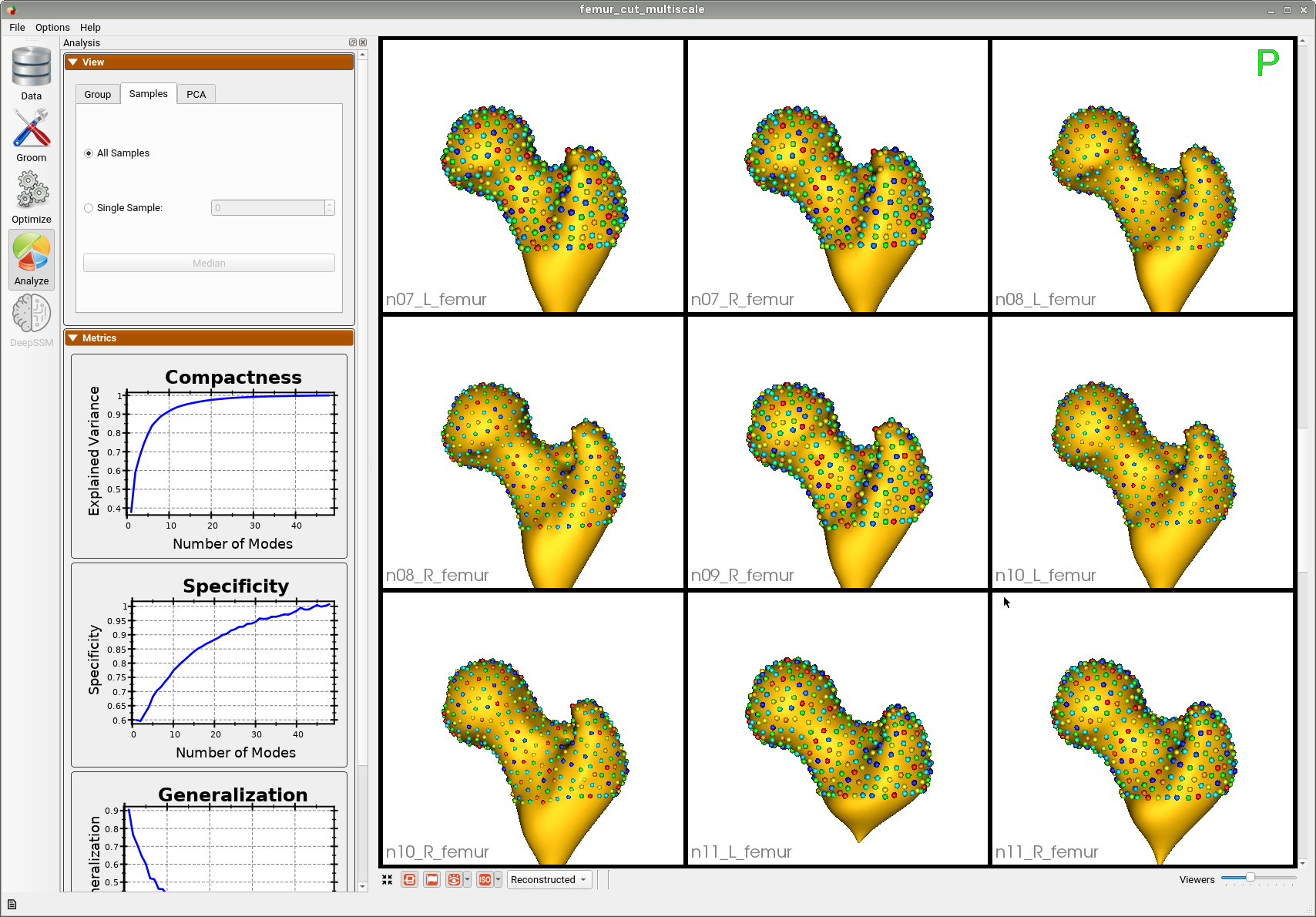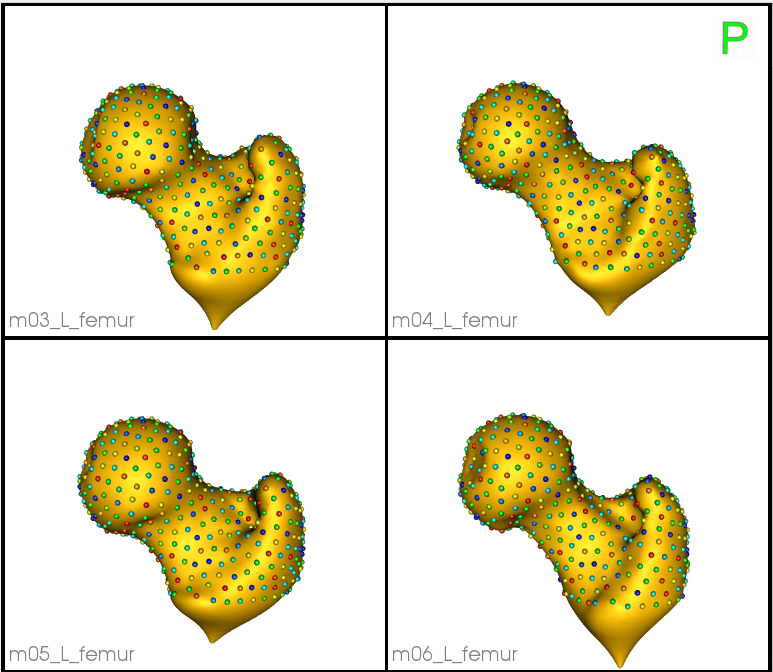# Femur: Shape Model from Meshes

## What and Where is the Use Case?

This use case demonstrates using ShapeWorks tools to perform the following.

• Build a shape model where shapes are given as triangular surface meshes
• Groom a dataset that contains both shapes (meshes) and their corresponding imaging data (e.g., CT)

The femur meshes in this data set have been segmented with various shaft lengths, as can be seen below. To remove this variability so that it is not captured in the shape model, the femurs are clipped using a cutting plane. The use case has a pre-defined cutting plane.The use case is located at: Examples/Python/femur.py

## Running the Use Case

Important

Minimum of 32GB of RAM required to run the full use case.

To run the use case, run RunUseCase.py (in Examples/Python/) with proper tags. The tags control the type of input data and the optimization method. See Getting Started with Use Cases for the full list of tags.

To run the full pipeline with multi-scale and the pre-defined cutting plane:

$cd /path/to/shapeworks/Examples/Python$ python RunUseCase.py femur


This calls femur.py (in Examples/Python/) to perform the following.

• Grooms the images and meshes. See Grooming Data for details about these preprocessing steps.
• Optimizes particle distribution (i.e., the shape/correspondence model) by calling optimization functions in OptimizeUtils.py (in Examples/Python/). See Optimizing Shape Model for details about algorithmic parameters for optimizing the shape model.
• Launches ShapeWorks Studio to visualize the use case results (i.e., the optimized shape model and the groomed data) by calling functions in AnalyzeUtils.py (in Examples/Python/).

If you wish to start with the optimization step using previously groomed data, add --skip_grooming tag.

$python RunUseCase.py femur --skip_grooming  To groom both the meshes and their corresponding images, use --groom_images tag. The image origin, size, and spacing will be used in mesh rasterization. $ python RunUseCase.py femur --groom_images


If this tag is not used, grooming will be done on meshes only. The origin and size will be inferred from the meshes, and isotropic spacing will be used unless the user specifies otherwise for rasterization.

## Grooming Data

We start with full unsegmented images (CT scans) of each femur's hip and segmented meshes in this use case. ShapeWorks needs a volumetric representation of shapes in the form of signed distance transforms to optimize the shape model. Hence, given surface meshes are first converted to image-based representation (i.e., binary segmentations). Additionally, the corresponding unsegmented images need to be carried through each grooming step with the meshes to be used for analysis. Each step's results are shown for the meshes (note every step is performed on both the meshes the images, although the resulting images are not shown here). For a description of the grooming tools and parameters, see: How to Groom Your Dataset?.

1. Reflect Meshes: In this use case, we often have both right and left femur surface meshes. To align all the femurs, we choose one side to reflect both the image and mesh.
2. Meshes to Volumes: Meshes must be turned into binary volumes using rasterization. The corresponding image origin, size, and spacing are used to generate the volume.
3. Isotropic Resampling: Both the image and rasterized segmentations are resampled to have an isotropic voxel spacing using a user-defined spacing. This step could also produce segmentations with smaller voxel spacing, thereby reducing aliasing artifacts (i.e., staircase/jagged surface) due to binarization.
4. Apply Padding: Segmentations that touch the image boundary will have an artificial hole at that intersection. Segmentations are padded by adding a user-defined number of voxels along each image direction (rows, cols, and slices) to avoid introducing artificial holes.
5. Center of Mass Alignment: This translational alignment step is performed before rigidly aligning the samples to a shape reference. This factors out translations to reduce the risk of misalignment and allow for a medoid sample to be automatically selected as the reference for rigid alignment.
6. Reference Selection: The reference is selected by first computing the mean (average) distance transform of the segmentations, then selecting the sample closest to that mean (i.e., medoid).
7. Rigid Alignment: All of the segmentations and images are then aligned to the selected reference using rigid alignment, which factors out the rotation and remaining translation. The alignment parameters are computed based on aligning segmentations and then applied to their corresponding images. The samples must be centered before they are aligned to a reference. This step can be performed with Isotropic Resampling as it is in the left atrium use case. In the Femur use case, we do so separately so that we can get the translation and apply it to the cutting plane if it has already been selected.
8. Clip Segmentations: Because the femur segmentations vary in shaft lengths, we use the defined cutting plane to clip them, so only the region of interest remains.
9. Cropping: The images and segmentations are cropped so that all of the samples are within the same bounding box. The bounding box parameters are computed based on the smallest bounding box that encapsulates all the given dataset segmentations.
10. Distance Transform: Finally, the signed distance transform is computed, and the dataset is now ready for the optimize phase.## Optimizing Shape Model

Below are the default optimization parameters when running this use case using the --use_single_scale tag. For a description of the optimize tool and its algorithmic parameters, see: How to Optimize Your Shape Model. Note that use_shape_statistics_after parameter is not used when --use_single_scale tag is given to the RunUseCase.py (in Examples/Python/).

$python RunUseCase.py femur --use_single_scale   "number_of_particles" : 1024, "use_normals": 0, "normal_weight": 10.0, "checkpointing_interval" : 200, "keep_checkpoints" : 1, "iterations_per_split" : 4000, "optimization_iterations" : 4000, "starting_regularization" : 100, "ending_regularization" : 0.1, "recompute_regularization_interval" : 2, "domains_per_shape" : 1, "domain_type" : 'image', "relative_weighting" : 10, "initial_relative_weighting" : 1, "procrustes_interval" : 1, "procrustes_scaling" : 1, "save_init_splits" : 1, "verbosity" : 2, "use_statistics_in_init" : 0  This use case can be run using the multi-scale optimization without the --use_single_scale tag as follows. $python RunUseCase.py femur


The use_shape_statistics_after parameter is used to trigger the multi-scale optimization mode.

        "use_shape_statistics_after": 64


## Analyzing Shape Model

ShapeWorks Studio visualizes/analyzes the optimized particle-based shape model by visualizing the mean shape, individual shape samples, and the shape modes of variations. For more information, see: How to Analyze Your Shape Model?.

Here is the mean shape of the optimized shape mode using single-scale optimization.

Here are femur samples with their optimized correspondences.Zooming in some femur samples.Here is a video showing the shape modes of variation (computed using principal component analysis - PCA) of the femur dataset using single-scale optimization.